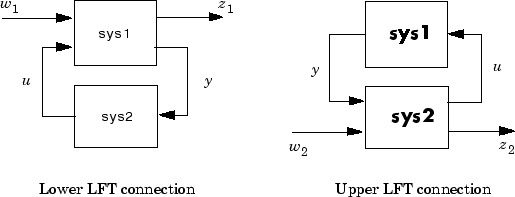Documentation

lft

Generalized feedback interconnection of two models (Redheffer star product)

Syntax

lft
sys = lft(sys1,sys2,nu,ny)

Description

lft forms the star product or linear fractional transformation (LFT) of two model objects or model arrays. Such interconnections are widely used in robust control techniques.

sys = lft(sys1,sys2,nu,ny) forms the star product sys of the two models (or arrays) sys1 and sys2. The star product amounts to the following feedback connection for single models (or for each model in an array).This feedback loop connects the first nu outputs of sys2 to the last nu inputs of sys1 (signals u), and the last ny outputs of sys1 to the first ny inputs of sys2 (signals y). The resulting system sys maps the input vector [w1 ; w2] to the output vector [z1 ; z2].

The abbreviated syntax

sys = lft(sys1,sys2)

produces:

• The lower LFT of sys1 and sys2 if sys2 has fewer inputs and outputs than sys1. This amounts to deleting w2 and z2 in the above diagram.

• The upper LFT of sys1 and sys2 if sys1 has fewer inputs and outputs than sys2. This amounts to deleting w1 and z1 in the above diagram.Limitations

There should be no algebraic loop in the feedback connection.

Algorithms

The closed-loop model is derived by elementary state-space manipulations.